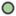# Scale Layer

(Redirected from Zoom Layer)
Jump to: navigation, searchLanguage: English## About Scale Layers

A "Scale Layer" scales the size of any primitive layers (regions, gradients, etc.) below it. "Scale Layer" is part of Transform Layers category.

## Parameters of Scale Layers

The parameters of the Scale Layers are:

 Name Value TypeAmount 1.000000 realOrigin 0.000000u,0.000000u vector

The Amount parameter may take the inverse of the exponential function (the natural log), as a raw value. For example:

• the natural log of 2.00 is 0.693147181, which up-scales to 200.0%
• the natural log of 1.00 is 0.0, which scales by 0.0%
• the natural log of 0.50 is -0.693147181, which down-scales to 50.0%
• the natural log of 0.10 is -2.302585092994045, which down-scales to 10.0%
• the natural log of 0.01 is -4.605170185988091, which down-scales to 1.0%

The Origin is the center of the scale.

``` In versions prior to 0.63.05 Scale Layer was called "Zoom layer".
```Language: English